Question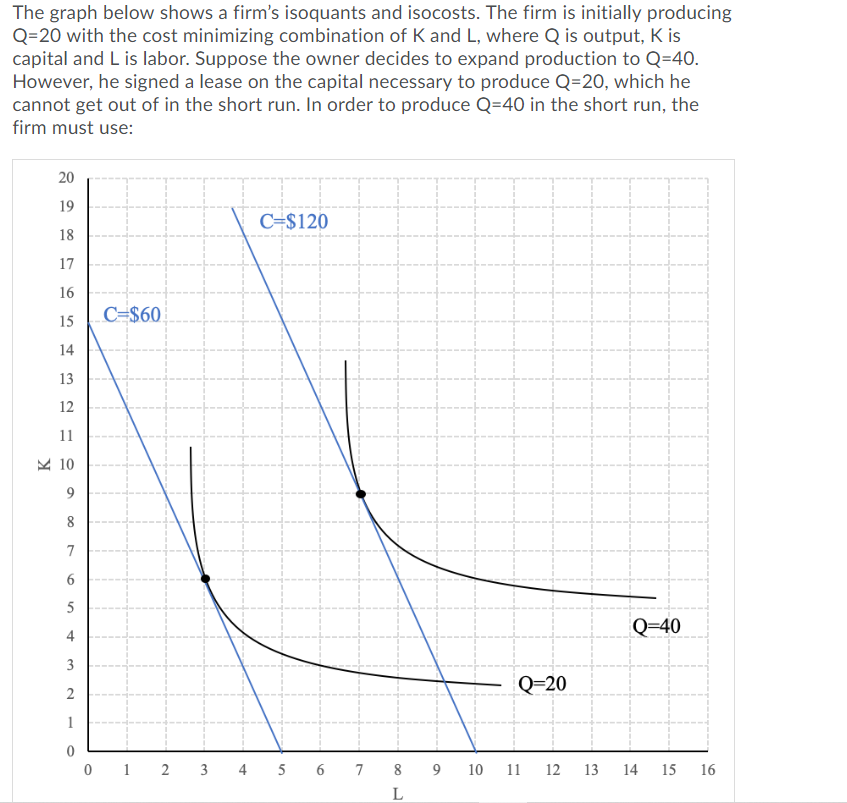A. L=3; K=6

B. L=10; K=6

C. L=7; K=9

D. L=8; K=7

B. L=10; K=6

(K is fixed at 6 units in the short run. So, the adjacent amount of L needed to produce 40 units is 10.)

#### Earn Coins

Coins can be redeemed for fabulous gifts.

Similar Homework Help Questions
• ### The graph below shows a firm's isoquants and isocosts. The firm is initially producing Q-20 with...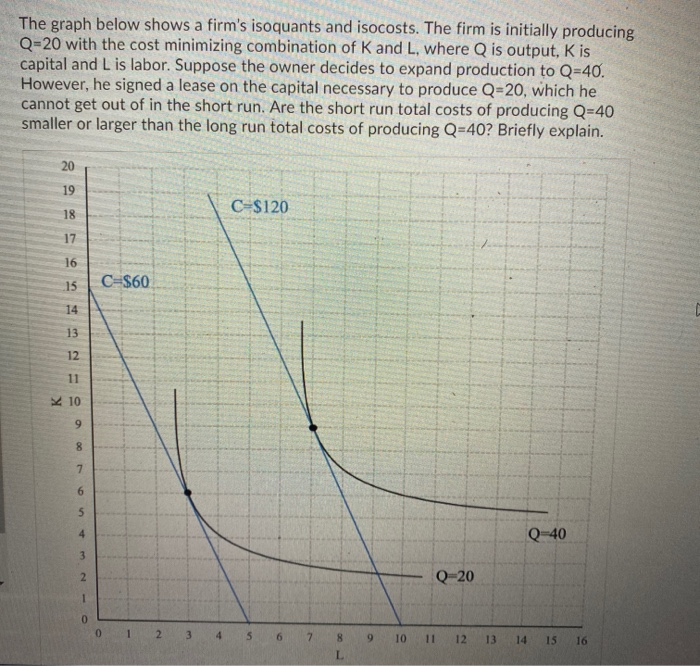The graph below shows a firm's isoquants and isocosts. The firm is initially producing Q-20 with the cost minimizing combination of K and L, where Q is output, Kis capital and Lis labor. Suppose the owner decides to expand production to Q=40. However, he signed a lease on the capital necessary to produce Q=20, which he cannot get out of in the short run. Are the short run total costs of producing Q=40 smaller or larger than the long run...

• ### The graph below shows a firm's isoquants and isocosts. The firm is initially producing Q=20 with...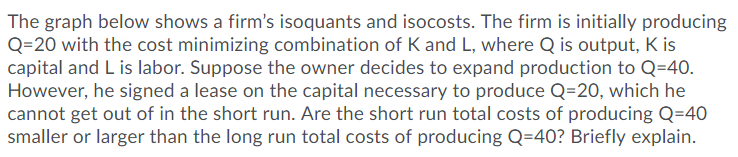The graph below shows a firm's isoquants and isocosts. The firm is initially producing Q=20 with the cost minimizing combination of K and L, where Q is output, Kis capital and L is labor. Suppose the owner decides to expand production to Q=40. However, he signed a lease on the capital necessary to produce Q=20, which he cannot get out of in the short run. Are the short run total costs of producing Q=40 smaller or larger than the long...

• ### The graph below shows a firm's isoquants and isocosts. The firm is initially producing Q-20 with...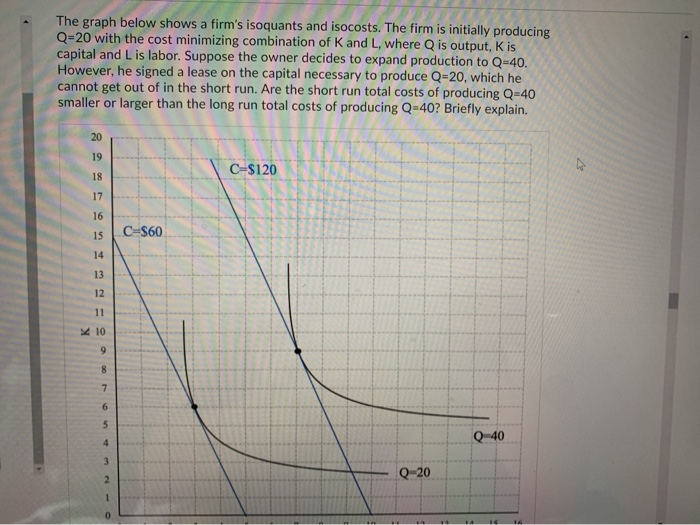The graph below shows a firm's isoquants and isocosts. The firm is initially producing Q-20 with the cost minimizing combination of K and L, where Q is output, Kis capital and Lis labor. Suppose the owner decides to expand production to Q=40. However, he signed a lease on the capital necessary to produce Q=20, which he cannot get out of in the short run. Are the short run total costs of producing Q-40 smaller or larger than the long run...

• ### 8. Suppose that the following graph shows a few of the isoquants for a firm's production...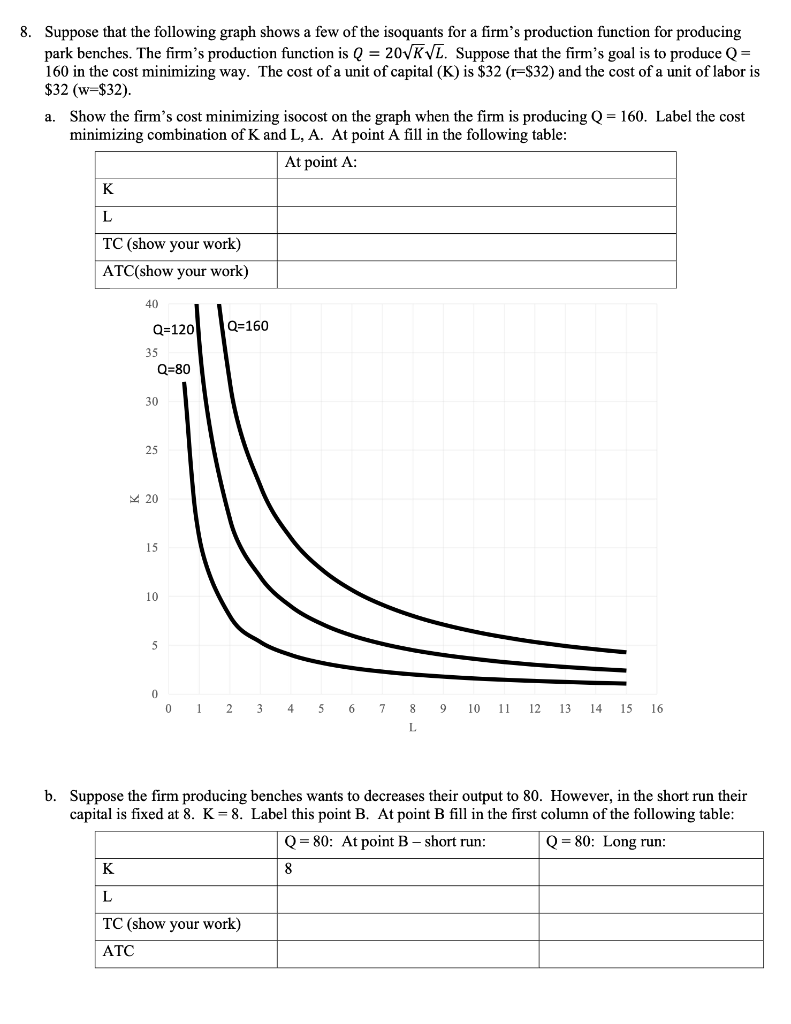8. Suppose that the following graph shows a few of the isoquants for a firm's production function for producing park benches. The firm's production function is Q = 20KVL. Suppose that the firm's goal is to produce Q= 160 in the cost minimizing way. The cost of a unit of capital (K) is \$32 (r=\$32) and the cost of a unit of labor is \$32 (w=\$32). a. Show the firm's cost minimizing isocost on the graph when the firm is...

• ### A. L=25; K=16 B. L=40; K=10 C. L=16; K=25 D. L=10; K=40 E. L=20; K=20 =...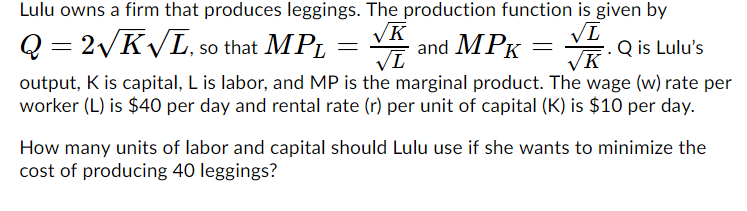A. L=25; K=16 B. L=40; K=10 C. L=16; K=25 D. L=10; K=40 E. L=20; K=20 = VE Lulu owns a firm that produces leggings. The production function is given by Q=2VKVL, so that MPL K and MPK = Q is Lulu's VL VK output, K is capital, L is labor, and MP is the marginal product. The wage (w)rate per worker (L) is \$40 per day and rental rate (r) per unit of capital (K) is \$10 per day. How...

• ### Let K be the level of capital in the short run. The prices of capital and labor are r = 10 and w-...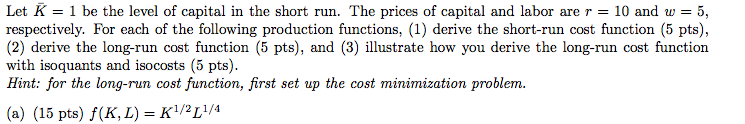Let K be the level of capital in the short run. The prices of capital and labor are r = 10 and w-5, respectively. For each of the following production functions, (1) derive the short-run cost function (5 pts), (2) derive the long-run cost function (5 pts), and (3) illustrate how you derive the long-run cost function with isoquants and isocosts (5 pts). Hint: for the long-run cost function, first set up the cost minimization problem. (a) (15 pts) f(K,...

• ### 6. From the production function given in the following table, draw a figure showing (a) the...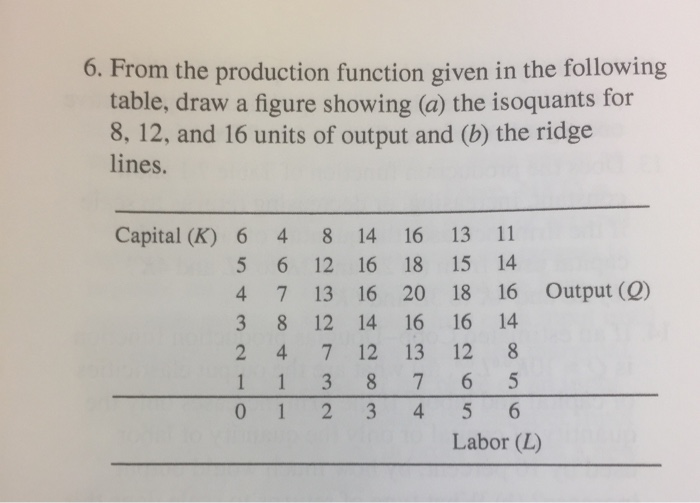6. From the production function given in the following table, draw a figure showing (a) the isoquants for 8, 12, and 16 units of output and (b) the ridge lines. Capital (K) 6 4 14 16 13 11 8 5 6 12 16 18 15 14 4 7 13 16 20 18 16 Output (Q) 16 16 14 3 8 12 14 2 47 12 13 12 8 5 3 7 6 1 1 6 1 2 3 4 5...

• ### 6. (10 points) Suppose a firm is facing the isoquants and isocosts from the graph below....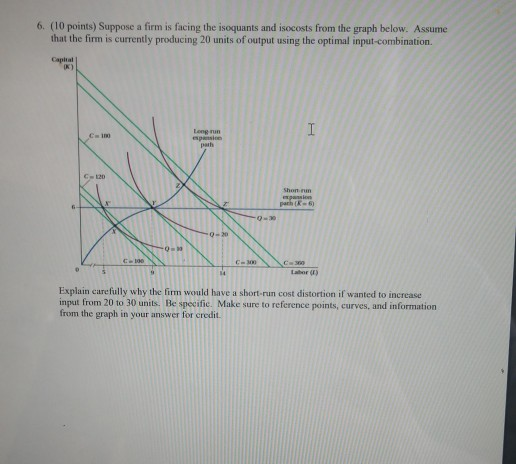6. (10 points) Suppose a firm is facing the isoquants and isocosts from the graph below. Assume that the firm is currently producing 20 units of output using the optimal input-combination Explain carefully why the firm would have a short-run cost distortion if wanted to increase input from 20 to 30 units. Be specific. Make sure to reference points, curves, and information from the graph in your answer for credit

• ### A. Q=4 B. Q=8 C. Q=10 D. Q=12 The graph below shows the average total cost...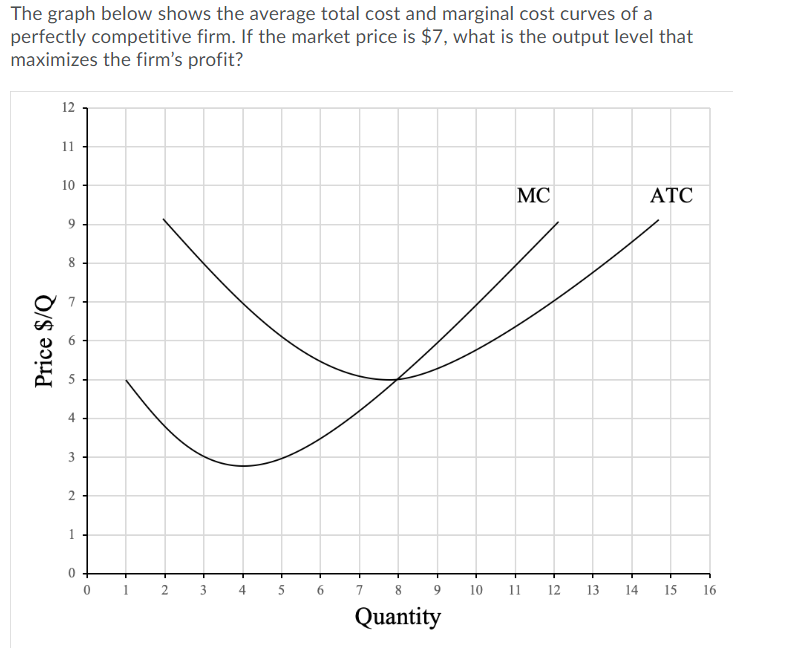A. Q=4 B. Q=8 C. Q=10 D. Q=12 The graph below shows the average total cost and marginal cost curves of a perfectly competitive firm. If the market price is \$7, what is the output level that maximizes the firm's profit? 12 11 10 MC ATC 9 8 Price \$/Q 4 3 2 0 2 3 4 5 9 10 11 12 دفا 14 15 16 6 7 8 Quantity

• ### 1. Imagine a firm has the following short-run production function: q=f(L,K) = K L – L?...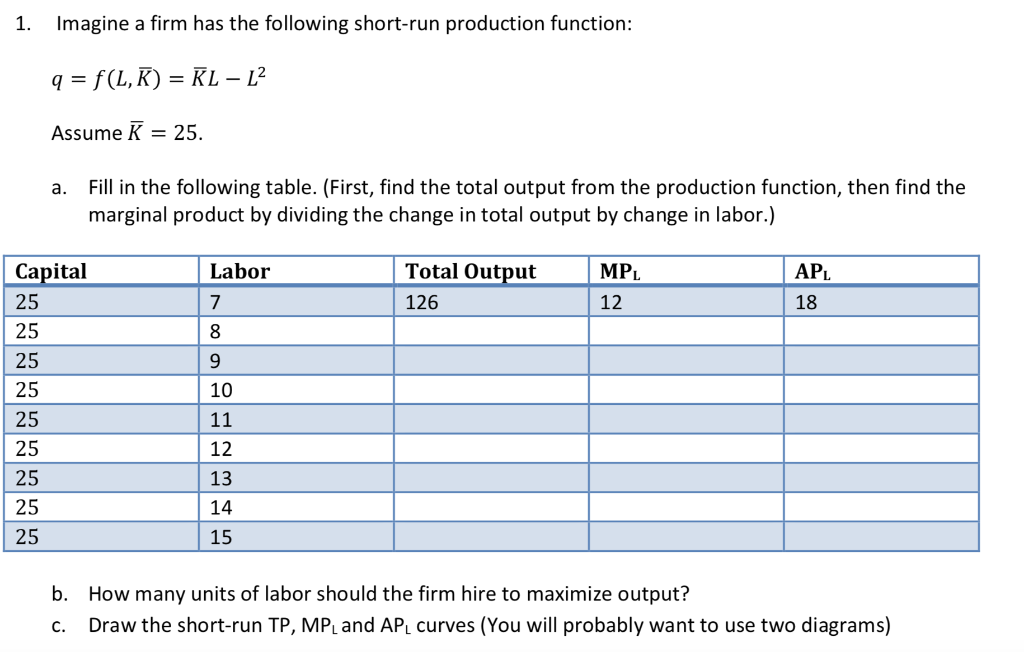1. Imagine a firm has the following short-run production function: q=f(L,K) = K L – L? Assume K = 25. a. Fill in the following table. (First, find the total output from the production function, then find the marginal product by dividing the change in total output by change in labor.) Capital MPL Labor 7 Total Output 126 APL 18 25 12 25 25 25 25 25 10 11 12 13 14 15 25 25 25 b. How many units...